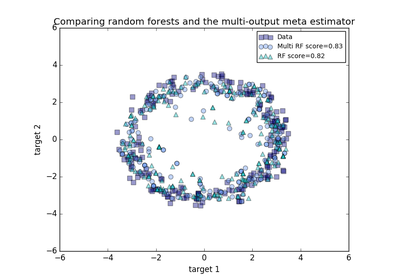# `sklearn.multioutput`.MultiOutputRegressor¶

class `sklearn.multioutput.``MultiOutputRegressor`(estimator, n_jobs=1)[source]

Multi target regression

This strategy consists of fitting one regressor per target. This is a simple strategy for extending regressors that do not natively support multi-target regression.

Parameters: estimator : estimator object An estimator object implementing fit and predict. n_jobs : int, optional, default=1 The number of jobs to run in parallel for fit. If -1, then the number of jobs is set to the number of cores. When individual estimators are fast to train or predict using n_jobs>1 can result in slower performance due to the overhead of spawning processes.

Methods

 `fit`(X, y[, sample_weight]) Fit the model to data. `get_params`([deep]) Get parameters for this estimator. `predict`(X) Predict multi-output variable using a model trained for each target variable. `score`(X, y[, sample_weight]) Returns the coefficient of determination R^2 of the prediction. `set_params`(\*\*params) Set the parameters of this estimator.
`__init__`(estimator, n_jobs=1)[source]
`fit`(X, y, sample_weight=None)[source]

Fit the model to data. Fit a separate model for each output variable.

Parameters: X : (sparse) array-like, shape (n_samples, n_features) Data. y : (sparse) array-like, shape (n_samples, n_outputs) Multi-output targets. An indicator matrix turns on multilabel estimation. sample_weight : array-like, shape = (n_samples) or None Sample weights. If None, then samples are equally weighted. Only supported if the underlying regressor supports sample weights. self : object Returns self.
`get_params`(deep=True)[source]

Get parameters for this estimator.

Parameters: deep : boolean, optional If True, will return the parameters for this estimator and contained subobjects that are estimators. params : mapping of string to any Parameter names mapped to their values.
`predict`(X)[source]
Predict multi-output variable using a model
trained for each target variable.
Parameters: X : (sparse) array-like, shape (n_samples, n_features) Data. y : (sparse) array-like, shape (n_samples, n_outputs) Multi-output targets predicted across multiple predictors. Note: Separate models are generated for each predictor.
`score`(X, y, sample_weight=None)[source]

Returns the coefficient of determination R^2 of the prediction.

The coefficient R^2 is defined as (1 - u/v), where u is the regression sum of squares ((y_true - y_pred) ** 2).sum() and v is the residual sum of squares ((y_true - y_true.mean()) ** 2).sum(). Best possible score is 1.0 and it can be negative (because the model can be arbitrarily worse). A constant model that always predicts the expected value of y, disregarding the input features, would get a R^2 score of 0.0.

Parameters: X : array-like, shape (n_samples, n_features) Test samples. y : array-like, shape (n_samples) or (n_samples, n_outputs) True values for X. sample_weight : array-like, shape [n_samples], optional Sample weights. score : float R^2 of self.predict(X) wrt. y.

Notes

R^2 is calculated by weighting all the targets equally using multioutput=’uniform_average’.

`set_params`(**params)[source]

Set the parameters of this estimator.

The method works on simple estimators as well as on nested objects (such as pipelines). The latter have parameters of the form `<component>__<parameter>` so that it’s possible to update each component of a nested object.

Returns: self :

## Examples using `sklearn.multioutput.MultiOutputRegressor`¶Comparing random forests and the multi-output meta estimator